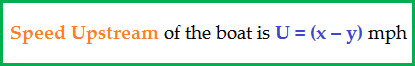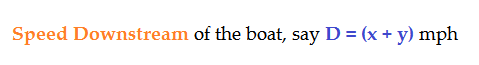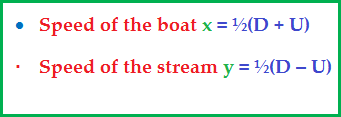## Boats And Streams

Problems on boats and streams are similar to those on Speed, Time and Distance.

The concepts of Relative Speed learnt in the lesson on Speed, Time and Distance find use here too.

Consider a boat rowing in a stream.

Let the boat row at ‘x’ mph and the stream flow at ‘y’ mph.

Now,

• When the boat rows against the stream flow:• When the boat rows down the stream flowFrom the above two formulas, we can also write:Let us solve a few questions now:

Example 1:

The speed of a boat upstream is 10mph and downstream 14 mph. What is the speed of the boat and speed of the stream?

Solution:

Speed Upstream, U = 10, and Speed Downstream D = 14

Speed of the boat, x = ½(D + U) = ½(14 + 10) = 12 mph

Speed of the stream, y = ½(D – U) = ½(14 – 10) = 2 mph.

Example 2:

A boat rows 20 miles upstream in 2 hours and the same distance downstream in 1hour. What is the stream speed?

Solution:

Speed = distance/time. So,

Speed downstream = 20/1 = 20 mph, and

Speed upstream = 20/2 = 10 mph.

Speed of the stream = ½(20 – 10) = 5 mph

Example 3:

A boat rows at 15 mph in still water. The boat takes twice as long to row up to the opposite bank as to row down the stream back. What is the stream speed?

Solution:

In traveling a same distance, speed and time are inversely proportional.

So, speed downstream = 2p mph, then

Speed upstream = p mph

Now, speed of the boat = ½(2p + p) = 15,

i.e. 3p = 30, p = 10 mph.

Therefore, D = 20 mph and U = 10 mph.

So, stream speed = ½(D – U) = ½( 20 – 10) = 5 mph